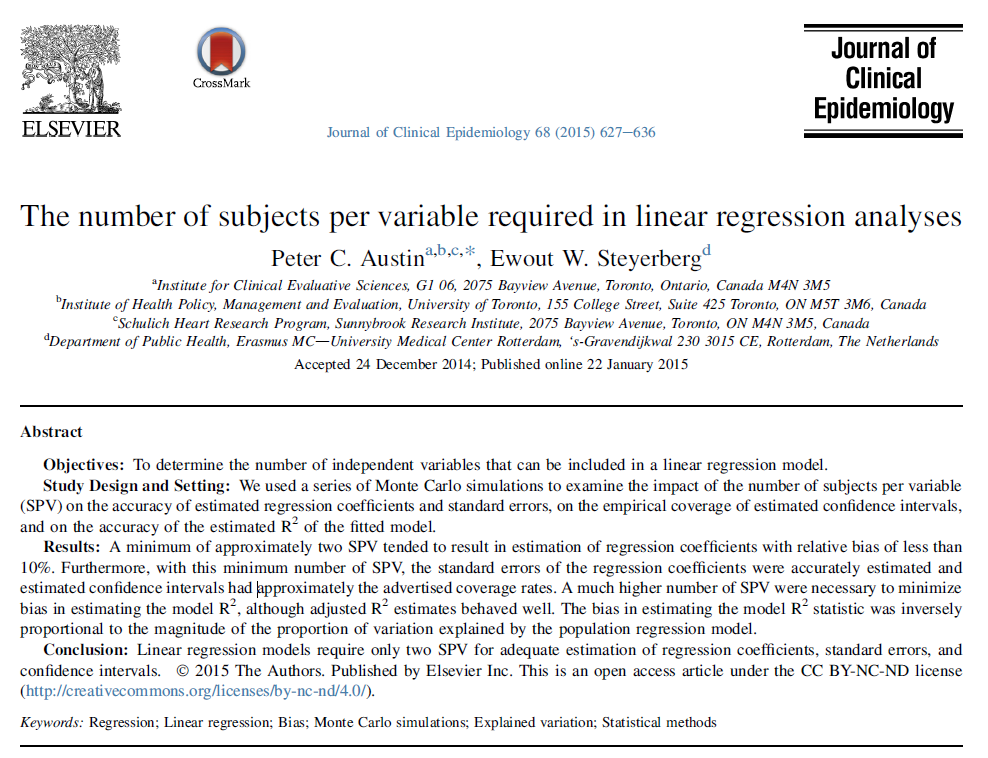# The number of subjects per variable required in linear regression analyses

## 2016-03-30

There are several rules of thumb out there about how many subjects that you need for a multiple linear regression model. Most of these rules look at the ratio of subjects per variable (SPV). If you have 100 subjects and 20 independent variables in your regression model

• then the SPV is 5. This article comes to the surprising conclusion that an SPV of 2 is just fine. In other words
• you could have 40 subjects and 20 independent variables and still be okay. This is independent of power considerations
• by the way
• but it still seems rather small to me. Read the paper yourself and let me know what you think.

<U+00A0>

Austin PC